# Step-by-Step Design Process for the MAX16833 High-Voltage High-Brightness LED Driver, Part 1

### Abstract

This application note details a step-by-step design process for the MAX16833 high-voltage high-brightness LED driver. This process can speed up prototyping and increase the chance for first-pass success. A typical design scenario is presented, along with example calculations based on the design constraints. Component selection trade-offs are discussed. A spreadsheet calculator is included to help calculate external component values. This application note focuses on the boost converter topology. However, the same process can be applied to other topologies as long as the underlying equations are understood. For a buck-boost converter design example, see application note 5659, “Step-by-Step Design Process for the MAX16833 High-Voltage High-Brightness LED Driver, Part 2.”

### Introduction

This application note is the first in a series that details a step-by-step design process for the MAX16833 high-voltage high-brightness LED driver to speed up prototyping and increase the chance for first-pass success. The MAX16833 is a peak current-mode-controlled LED driver, capable of driving an LED string in several different architectures: boost, buck-boost, SEPIC, flyback, and high-side buck topologies. The second application note in the series, application note 5659, “Step-by-Step Design Process for the MAX16833 High-Voltage High-Brightness LED Driver, Part 2,” focuses on the buck-boost converter topology. This application note, Part 1, focuses on the boost topology.
The MAX16833 offers several features: a dimming driver designed to drive an external p-channel MOSFET, extremely fast PWM current switching to the LEDs without transient overvoltage or undervoltage, analog dimming, programmable switching frequency between 100kHz and 1MHz, and the option of either a ramp output for frequency dithering or a voltage reference for precisely setting the LED current with few external components.
For the design example here in Part 1, a 7 LED string is driven with a constant current of 1A. Assume that each LED has a typical forward voltage drop of 3V and a dynamic resistance of 0.2Ω. Also assume that the LED driver circuit is running directly off of the car battery, which has a typical voltage of 12V but can vary from 6V to 16V. Since the LED string voltage is always greater than the input voltage, the boost configuration is chosen.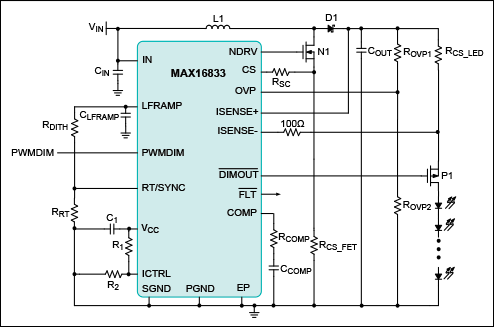Figure 1. Typical operating circuit.

### Inductor Selection (Boost)

In order to select the right inductor value, the maximum duty cycle must be calculated:(Eq. 1)
Where VLED is the forward voltage of the LED string in volts, VD is the forward drop of the rectifying diode (approximately 0.6V), VINMIN is the minimum input-supply voltage in volts, and VFET is the average drain-to-source voltage of the switching MOSFET in volts when it is on (assume 0.2V initially).
The maximum duty cycle and LED current determine the average inductor current.(Eq. 2)
The peak inductor current is defined as follows:(Eq. 3)
Where ΔIL is the peak-to-peak inductor current ripple in amperes.
Finally, the minimum inductor value can be calculated:(Eq. 4)
Below is a numerical example based on the design problem outlined in the Introduction. Choose an inductor current ripple of 50%. Lower ripple current would require a larger (and typically more expensive) inductor. Higher ripple current requires more slope compensation and increased input capacitance.(Eq. 5)(Eq. 6)(Eq. 7)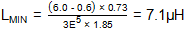(Eq. 8)
Once the minimum inductor value has been determined, a real inductor value must be chosen that is as close to LMIN as possible without going under. Recalculate the peak inductor current and ripple using the chosen inductor value. These numbers are necessary for additional calculations going forward.(Eq. 9)(Eq. 10)(Eq. 11)
Ensure that the chosen inductor has a current rating higher than ILP. Typically, 20% headroom is used for inductor peak current.

### Input Capacitor Selection

In a boost converter, the input current is continuous so the RMS ripple current is low. Both bulk capacitance and ESR contribute to the input ripple. Assume equal ripple contributions from bulk capacitance and ESR if aluminum electrolytic and ceramic capacitors are both used in parallel. If only ceramic capacitors are used, most of the input ripple comes from the bulk capacitance (since ceramic capacitors have very low ESR). Use the equations below to calculate the minimum input bulk capacitance and maximum ESR: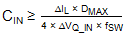(Eq. 12)
Where ΔVQ_IN is the portion of input ripple due to the capacitor discharge.(Eq. 13)
Where ΔVESR_IN is the input ripple due to ESR.
Assume that a maximum of 120mV of input ripple can be tolerated (2% of VINMIN). Also, assume that 95% of this input ripple comes from the bulk capacitance. This assumption may need to be revisited if the calculated values are not easily attained with actual components. Based on the stated design specifications, the input capacitor is calculated as follows:(Eq. 14)(Eq. 15)
Use two 4.7µF capacitors in parallel to achieve the 8.5µF minimum bulk capacitance. Ensure that the chosen capacitors meet the minimum bulk capacitance requirement at the operating voltage (capacitance can decrease substantially with a change in voltage in ceramic capacitors).

### Output Capacitor Selection

The purpose of the output capacitor is to reduce the output ripple and source current to the LEDs when the switching MOSFET is on. Both bulk capacitance and ESR contribute to the total output voltage ripple. If ceramic capacitors are used, a majority of the ripple comes from the bulk capacitance. Use Equation 16 to calculate the required bulk capacitance:(Eq. 16)
Where ΔVQ_OUT is the portion of output ripple due to the capacitor discharge.
The remaining ripple, ΔVESR_OUT, comes from the output capacitor ESR, which can be calculated as follows: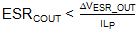(Eq. 17)
To determine the total allowed output ripple, multiply the allowed LED current ripple by the dynamic impedance of the LED string. The dynamic impedance of an LED is defined as ΔV/ΔI at the operating LED current and can be determined from the I-V curve in the LED data sheet. If an I-V curve is not provided in the LED data sheet, then it must be measured manually.
Use multiple ceramic capacitors in parallel to reduce the effective ESR and ESL of the bulk output capacitance.
During PWM dimming, the ceramic output capacitors might cause some audible noise. To reduce this noise, use an electrolytic or tantalum capacitor in conjunction with the ceramic capacitors to provide most of the bulk capacitance necessary. A low acoustic noise ceramic capacitor can also be used.1
Assume a maximum LED current ripple of 0.1 × ILED. Also, assume that the dynamic impedance of the chosen LED is 0.2Ω (1.4Ω total for the 7 LED string). The total output voltage ripple is then calculated as follows:
 VOUTRIPPLE = 0.1A × 1.4Ω = 140mV (Eq. 18)
Assuming a ripple contribution of 95% from bulk capacitance, the output capacitor is calculated as follows:(Eq. 19)(Eq. 20)
Use four 4.7µF capacitors in parallel to achieve the minimum output capacitance of 18.3µF. Ensure that the chosen capacitors meet the minimum bulk capacitance requirement at the operating voltage (capacitance can decrease substantially with a change in voltage in ceramic capacitors).

### Overvoltage Protection

If the LEDs are open, the converter tries to increase the output voltage to achieve the desired LED current. This means that the output voltage could approach unsafe levels. The OVP input is provided to sense an overvoltage condition and limit the output voltage. In the event that VOVP exceeds 1.23V, NDRV is forced low until VOVP discharges to 1.16V.(Eq. 21)
For this design example, assume that a VOV of 42V is acceptable. Choose ROVP2 to be 10kΩ, then(Eq. 22)

### Current Sensing

The MAX16833 is a current-mode-controlled LED driver, which means that information about the inductor current and LED current is fed back into the loop.

### LED Current Sensing

The LED current is programmed by either a series high-side current-sense resistor or the voltage applied to the ICTRL input.
If VICTRL > 1.23V, the internal reference regulates the voltage across RCS_LED (VISENSE+ - VISENSE-) to 200mV. Therefore, the current-sense resistor RCS_LED sets the LED current.(Eq. 23)
If VICTRL < 1.23V, then the LED current is determined by RCS_LED and VICTRL. This allows the LEDs to be dimmed with an analog voltage.(Eq. 24)
Notice that when VICTRL = 1.23V, both equations are the same.
RCS_LED is also used to detect a short circuit across the LED string. If the voltage across ISENSE+ and ISENSE- exceeds 300mV for ≥ 1µs, then the short-circuit protection within the IC activates.

### Switching FET Current Sensing and Slope Compensation

At duty cycles greater than 50%, a load transient can cause subharmonic oscillation and loop instability without slope compensation. To keep the loop stable, add a resistor (RSC from CS to the source of the switching MOSFET). Internal to the MAX16833, there is a current source that feeds current through RSC to create a voltage VSC. This voltage is added to the voltage across RCS_FET and the result is compared to a reference.
 VCS = VSC + VCS_FET (Eq. 25)
The minimum amount of slope-compensation voltage needed to maintain stability is:
 VSCMIN = 0.5 × (inductor current downslope - inductor current upslope) × RCS_FET (Eq. 26)
The FET current-sense resistor, RCS_FET, has both the switching MOSFET current and the slope compensation current flowing through it.Figure 2. Slope compensation.
The slope-compensation voltage is defined as follows:(Eq. 27)
In order to calculate the minimum necessary slope-compensation voltage, assume the minimum supply voltage and minimum inductor value:(Eq. 28)(Eq. 29)
Therefore:(Eq. 30)
The factor of 1.5 is included to provide adequate margin.(Eq. 31)
Once RCS_FET has been determined, RSC can be calculated as follows:(Eq. 32)
Based on the stated design specifications, the slope compensation and current-sense resistors are calculated as follows:(Eq. 33)(Eq. 34)
The closest standard resistor value without going under is 68mΩ.(Eq. 35)

### Error Amplifier Compensation

In the boost configuration, the switching converter has a right-half-plane (RHP) zero that causes the loop to be unstable. The goal of loop compensation is to ensure that there is less than 180° of phase shift for loop gains > 0dB (and adequate phase margin). By adding a left-half-plane (LHP) pole, the loop gain can be rolled off to 0dB at approximately 1/5 fZRHP and the instability caused by the RHP zero can be avoided. The error amplifier must be compensated to ensure loop stability over all expected variations in operating conditions. The worst case RHP zero frequency is calculated as follows:(Eq. 36)
There is also a pole at the output of the switching converter. The output pole, fP2, can be calculated as follows:(Eq. 37)
Where COUT is the bulk output capacitance calculated above and ROUT is the effective output impedance.(Eq. 38)
Where RLED is the dynamic impedance of the LED string at the operating current in ohms.
The loop is compensated by adding a series resistor and capacitor (RCOMP and CCOMP) from COMP to SGND. RCOMP sets the crossover frequency and CCOMP sets the integrator zero frequency. For optimum performance, use the following equations:(Eq. 39)(Eq. 40)
Following the design example:(Eq. 41)(Eq. 42)(Eq. 43)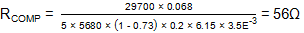(Eq. 44)(Eq. 45)

### PWM Dimming

Although analog dimming can be controlled by sweeping the voltage on ICTRL between 0V and 1.23V, sometimes it is desirable to dim the LEDs without changing the LED current. The MAX16833 allows for PWM dimming with a PWMDIM input and a %-overbar_pre%DIMOUT%-overbar_post% output.
The MAX16833 is designed to drive a high-side p-channel MOSFET. By dimming with a high-side p-channel MOSFET instead of a low-side n-channel MOSFET, one less connection is needed from the MAX16833 board to the LEDs. Figure 3 shows a generic MAX16833 solution needing only three connections to create a boost or buck-boost LED driver.Figure 3. Three-terminal MAX16833 solution.
The MAX16833 is designed for front light assemblies and therefore is only suited for applications where less than 500:1 dimming is required.
To maximize the possible dimming ratio, several things can be done:
• Use a slow dimming frequency. The human eye is typically incapable of distinguishing dimming ratios greater than 100Hz.
• Increase the switching frequency. This has the added benefit of reducing the necessary size of the power components. However, this decreases efficiency.
• Decrease the inductor value. This increases the inductor ripple current, which increases radiated emissions and decreases efficiency.
Note: At very slow dimming frequencies (e.g., 1Hz turn signal), careful consideration must be made to prevent the output of the boost converter from discharging to within 1.5V of the battery. This is because a short across the LEDs is detected by sensing the voltage difference between VISENSE+ and VIN. If VISENSE+ falls to within 1.5V of the battery voltage, then the %-overbar_pre%FLT%-overbar_post% output asserts low, erroneously indicating that a fault has occurred. The ISENSE+ input has a typical bias current of 200µA, which can discharge COUT during the off phase of the PWMDIM signal. The OVP resistor-divider is also a leakage path that can discharge the output capacitor (see Figure 4).Figure 4. Output capacitor leakage paths.

### Frequency Dithering

The MAX16833/MAX16833C feature an LFRAMP output that simplifies frequency dithering of the internal oscillator (spread spectrum). Consider using this feature when the design has stringent EMI requirements. LFRAMP outputs a triangle wave between 1V and 2V with a frequency set by a single bypass capacitor.(Eq. 46)
fLFRAMP should be slower than fSW by at least a factor of 10.
Assuming a dithering frequency of 500Hz, CLFRAMP can be calculated as follows:(Eq. 47)
To dither the frequency of the internal oscillator, connect a resistor between LFRAMP and RT/SYNC.Figure 5. Not using LFRAMP.Figure 6. Using LFRAMP to dither the internal oscillator frequency.
The variation in oscillator frequency is determined by RDITH.(Eq. 48)(Eq. 49)
Figure 7 demonstrates the effect of frequency dithering on the internal oscillator.Figure 7. LFRAMP in action.
Choose RRT and RDITH such that the internal oscillator operates between 100kHz and 1MHz.
Assume that a ΔfSW of 12.5% is desired.(Eq. 50)Figure 8. Output spectral content.

### Proper Layout

Along with dithering, proper layout is important for good EMI performance. The key to minimizing EMI due to layout is to identify the discontinuous current paths.Figure 9. Simplified schematic.
Figure 10 shows current versus time for some of the external components. The high di/dt occurrences are circled in orange.Figure 10. Various current waveforms.Figure 11. High di/dt paths critical to layout.
In order to improve EMI, keep the components highlighted in red as close to each other as possible. Keep the traces between these components as short as possible to reduce parasitic inductance along the high di/dt paths.

### Other EMI Design Considerations

If additional EMI improvements are needed after frequency dithering and layout optimization, a few other design techniques can be used. EMI can be reduced by slowing down the rise and fall times of the LX node. The two most common ways of doing this are to add a small gate resistor to N1 or a small ferrite bead to the drain of N1. Either of these additions somewhat improves the EMI but at the cost of reducing efficiency.

### Conclusion

The complete boost LED driver schematic is shown in Figure 12. By following the step-by-step design process outlined in this application note, significant time can be saved during the debug and test phase of the project.Figure 12. Typical application circuit based on example calculations.
For additional details, refer to the MAX16833 data sheet and the MAX16833EVKIT data sheet.
For information on the design process focused on the buck-boost converter topology of the MAX16833, see application note 5659, “Step-by-Step Design Process for the MAX16833 High-Voltage High-Brightness LED Driver, Part 2.”

#### References

1. For information on acoustic noise and capacitors for acoustic noise reduction, see www.murata.com/products/capacitor/solution/naki.html.